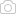Updated: 9/2/2020

# Measures of Association

0%
Topic
Review Topic
0
0
Topic
Overview
• Measures of association quantify relationships between variables
• In medicine and public health, measures of association most often quantify the association of exposure with disease
• Measures of association can be presented and framed in different ways for diverse purposes
• 2x2 tables can be helpful in calculating these measures• note: these tables are slightly different from 2x2 tables used to calculate diagnostic testing parameters
 Relative Risk, Odds Ratio, Attributable Risk, and Absolute Risk Reduction Relative risk (RR) risk of disease in exposed group/risk of disease in unexposed group = [a/(a+b)] / [c/(c+d)] used in cohort studies or other studies where total population is known RR > 1 exposure is associated with increased risk of disease RR < 1 exposure is associated with decreased risk of disease RR = 1 no association between exposure and disease Odds ratio (OR) odds of having disease in exposed group/odds of having disease in unexposed group  = ad/bc primarily used in case control studies  can be used in cohort studies when outcome is rare OR approximates RR for rare outcomes OR > 1 odds of developing disease are greater in exposed group OR < 1 odds of developing disease are reduced in exposed group OR = 1 odds of developing disease are equal in exposed and unexposed group Attributable risk (AR) "How much greater risk is present in the exposed group than the unexposed?" risk of disease in exposed group - risk in unexposed group = a/(a+b) - c/(c+d) Attributable risk percent (ARP) "What percent greater risk is present in the exposed group than the unexposed?" (risk of disease in exposed group - risk in unexposed group)/risk in exposed group = [a/(a+b) - c/(c+d)] / [a/(a+b)] Absolute risk reduction (ARR)  "How much risk is reduced by the intervention (or exposure)?" risk in control group - risk in intervention group = c/(c+d) - a/(a+b) Number Needed to Treat and Number Needed to Harm Number needed to treat (NNT) "How many patients needed to be treated before 1 patient will benefit?" e.g., if a treatment affords a 25% ARR, generally 4 people will have to be treated for every 1 that benefits NNT calculation is the inverse of ARR  =1/[c/(c+d) - a/(a+b)] Number needed to harm (NNH) "How many patients need to be exposed before one patient will be harmed?" NNH calculation is the inverse of AR = 1/[a/(a+b) - c/(c+d)] Population-Level Measures of Association Used to make public health decisions and allocate resources Population attributable risk (PAR) "What amount of the risk of disease in a population is attributable to a specific exposure?" (as opposed to baseline population risk) total population incidence of disease - incidence of disease amongst unexposed  = [(a+c)/(a+b+c+d) - (c/c+d)]  unit is per person e.g., if your PAR for obesity on heart disease is 0.004, the risk of heart disease for the total population that is likely due to obesity is 4 cases per 1,000 people if obesity was eliminated from the population, 4 cases of cardiac disease per every 1,000 people would be eliminated Population attributable risk percent (PAR%) "What percent of disease cases amongst a population can be attributed to a specific exposure?" (as opposed to baseline population risk) [(total population incidence of disease - incidence of disease amongst unexposed)/total population incidence of disease] * 100 = [(a+c)/(a+b+c+d) - (c/c+d)]/[(a+c)/(a+b+c+d)] * 100